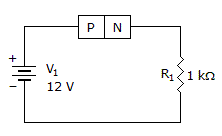# Electronics - Semiconductor Principles

### Exercise :: Semiconductor Principles - General Questions

21.

How many valence electrons are in every semiconductor material?

 A. 1 B. 2 C. 3 D. 4

Answer: Option D

Explanation:

No answer description available for this question. Let us discuss.

22.

What is a type of doping material?

 A. extrinsic semiconductor material B. pentavalent material C. n-type semiconductor D. majority carriers

Answer: Option B

Explanation:

No answer description available for this question. Let us discuss.

23.

Minority carriers are many times activated by:

 A. heat B. pressure C. dopants D. forward bias

Answer: Option A

Explanation:

No answer description available for this question. Let us discuss.

24.

What is the voltage across R1 if the P-N junction is made of silicon?A. 12 V B. 11.7 V C. 11.3 V D. 0 V

Answer: Option C

Explanation:

No answer description available for this question. Let us discuss.

25.

If conductance increases as temperature increases, this is known as a:

 A. positive coefficient B. negative current flow C. negative coefficient D. positive resistance

Answer: Option C

Explanation:

A negative temperature coefficient (NTC) occurs when the thermal conductivity of a material rises with increasing temperature, typically in a defined temperature range.

Note: The reciprocal of electrical Resistance is called Conductance.

#### Current Affairs 2021

Interview Questions and Answers